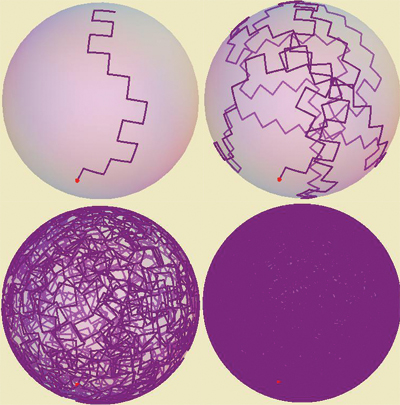# Rolling a random walk on a sphere

A ball rolls down an inclined plane, encountering horizontal obstacles, at which it rolls left/right with equal probability. There are regularly spaced staggered gaps that let the ball roll down to the next, lower obstacle. The pattern resembles a binary tree:Suppose the vertical and horizontal rolls have equal length $\delta$. Tracing out the roll contact point on the ball surface we see a random walk, with each step a geodesic arc of length $\delta$, and $90^\circ$ turns. I expected that for rational (multiples of $\pi$) $\delta$, the trace would not fill the surface, but the experiment below for $\delta=\pi/16$ (for 10, $10^2$, $10^3$, $10^4$ downhill steps) indicates otherwise.

For which $\delta$ will this trace fill the sphere surface?Thanks for any insights!

Answer: The surface will be filled for every $\delta$ except $\pi/2$ and $\pi$. See Scott Carnahan's answer below, and Dylan Thurston's simplification. I find this answer remarkable!

• There was a related problem about the density of a group generated by two spherical rotations, both of angular measure pi/8. I thought the group was finite, but turned out not to be. I suspect Bill Thurston's answer there may be relevant here. If I recall the link I will post it. Gerhard "Too Many Comments To Remember" Paseman, 2011.04.30 May 1, 2011 at 2:35
• Here it is: "Maneuvering with limited moves on $S^2$, mathoverflow.net/questions/62156 . You are right, Gerhard, it does seem related... May 1, 2011 at 3:09
• Given that the finite subgroups of $SO(3)$ follow the ADE classification, it shouldn't be too surprising that you are restricted to a very small class of admissible rotations. May 1, 2011 at 5:15
• I would just like to take this opportunity to say I absolutely love your questions. May 2, 2011 at 19:31
• @BB: I am truly honored! :-) May 2, 2011 at 21:23

Let $A = \begin{pmatrix} \cos \delta & -\sin \delta & 0 \\ \sin \delta & \cos \delta & 0 \\ 0 & 0 & 1 \end{pmatrix}$, and let $B = \begin{pmatrix} 1 & 0 & 0 \\ 0 & \cos \delta & -\sin \delta \\ 0 & \sin \delta & \cos \delta \end{pmatrix}$ be rotation by $\delta$ along the $z$ and $x$ axes, respectively. In suitable coordinates, a progression down one step in the tree is either $AB$ or $AB^{-1}$.

The trace will fill (a.s.) a dense subset of the surface if and only if the closure of the group generated by $AB$ and $AB^{-1}$ is not a subgroup of $SO(3)$ of dimension zero or one.

The dimension zero closed subgroups of $SO(3)$ are either cyclic, dihedral, or symmetries of Platonic solids, and the dimension one closed subgroups are conjugates of $SO(2)$ and $O(2)$. Therefore, it suffices to determine which values of $\delta$ yield a pair of elements in either a conjugate of $O(2)$, or a conjugate of one of the three Platonic groups (isomorphic to $A_4$, $S_4$, and $A_5$).

In order for $AB$ and $AB^{-1}$ to both lie in a conjugate of $O(2)$ it is necessary and sufficient that they have a common eigenvector with eigenvalue $\pm 1$ - this eigenvector is the axis of rotation. Writing this requirement explicitly yields a polynomial identity in $\sin \delta$ and $\cos \delta$ (whose solutions I haven't enumerated yet). Edit: Some straightforward case elimination with the $z$ coordinate of a common eigenvector shows that $\delta$ must be an integer multiple of $\pi/2$.

For the Platonic solutions, we can narrow down the solution set using the criterion that the rotation $(AB^{-1})^{-1}(AB) = B^2$ lies in the group, and Platonic solids have rotational symmetries of order at most 5. This means $\delta$ is a multiple of $\pi/3$, $\pi/4$ or $\pi/5$.

Since the traces of $AB$ and $AB^{-1}$ are both $(\cos \delta)(2 + \cos \delta)$, we can compare with character table entries to see if that number is the trace of an element in a Platonic group. It was pretty easy to eliminate candidates by eyeball in SAGE.

Conclusion: The only values of $\delta$ where the image is not dense are $0$, $\pm \pi/2$, and $\pi$.

• Beautifully clear reasoning, and a remarkable conclusion! May 1, 2011 at 11:08
• You can make the calculations slightly easier by cutting the step size in two. Let A be the matrix you wrote down, and let C by rotation by π/2 around the y axis. Then you can alternately take the steps to be AC and AC−1. The trace of AC is then just $cos\delta$. If we have a Platonic solid group, this must be 1+2cosα for α∈{±2π/3,±π/2,±2π/5,±4pi/5}. (Every element in a Platonic group is a rotation by one of these angles.) 2δ is also in this set, and it's easy to find the solutions by hand, using $\cos\delta=(\cos\alpha-1)/2$. May 1, 2011 at 11:36